### IMO Shortlist 1982 problem 14

Kvaliteta:
Avg: 0.0
Težina:
Avg: 0.0
Let$ABCD$ be a convex plane quadrilateral and let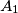$A_1$ denote the circumcenter of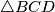$\triangle BCD$. Define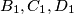$B_1, C_1,D_1$ in a corresponding way.

(a) Prove that either all of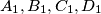$A_1,B_1, C_1,D_1$ coincide in one point, or they are all distinct. Assuming the latter case, show that$A_1$, C1 are on opposite sides of the line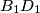$B_1D_1$, and similarly,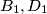$B_1,D_1$ are on opposite sides of the line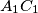$A_1C_1$. (This establishes the convexity of the quadrilateral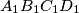$A_1B_1C_1D_1$.)

(b) Denote by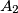$A_2$ the circumcenter of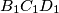$B_1C_1D_1$, and define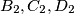$B_2, C_2,D_2$ in an analogous way. Show that the quadrilateral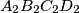$A_2B_2C_2D_2$ is similar to the quadrilateral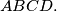$ABCD.$
Source: Međunarodna matematička olimpijada, shortlist 1982